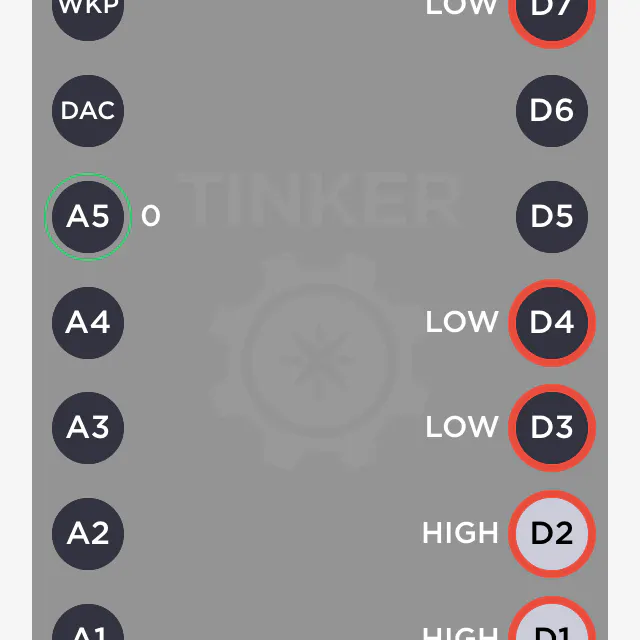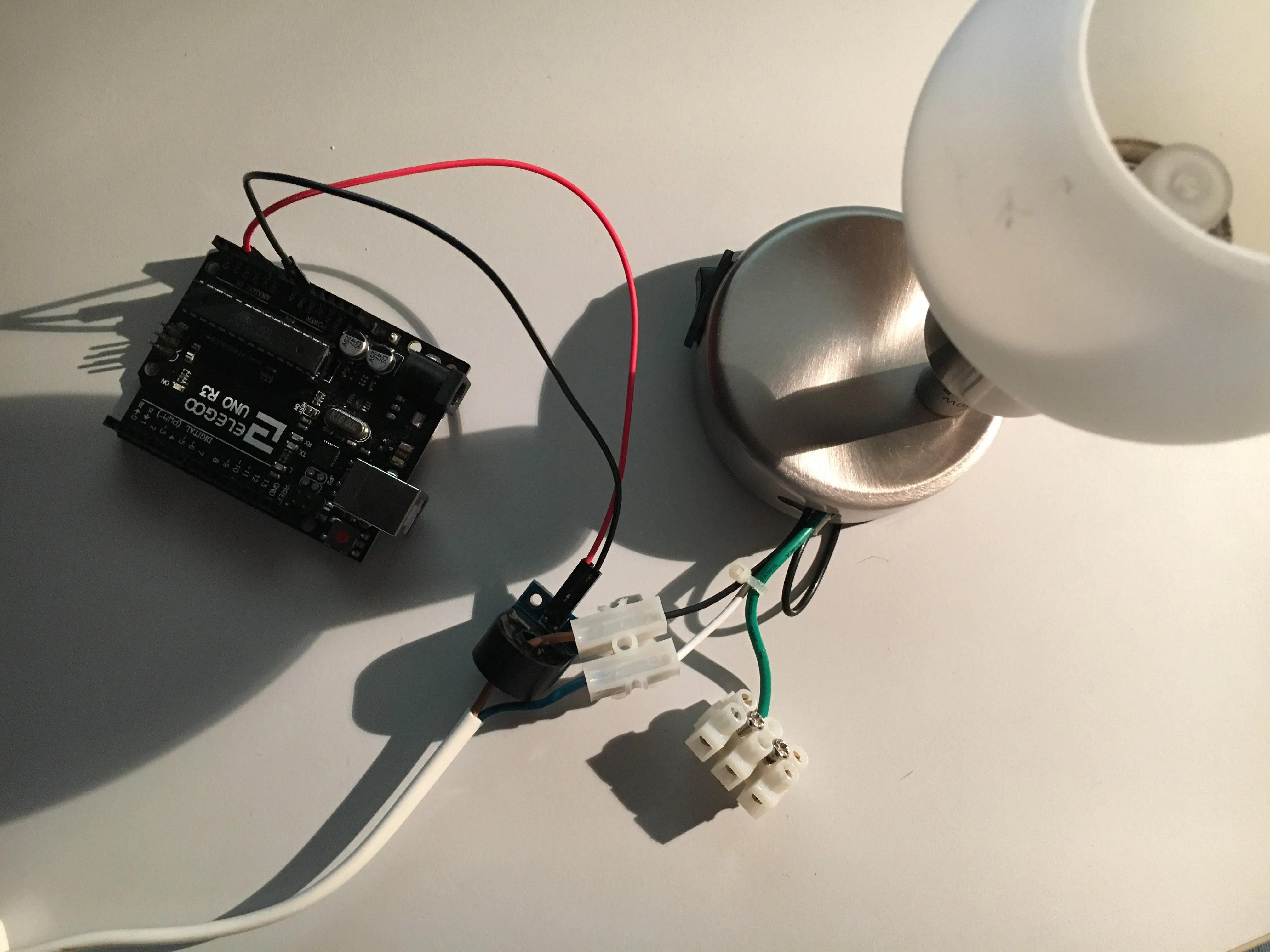# AC Current Sensor

Anyone thinking of an Australian hard-rock band at AC/DC is absolutely right here.

BeginnerProtip1 hour36,604## Things used in this project

### Hardware componentsArduino UNO
×1Jumper wires (generic) 2 pieces: male2female
×1
 AC Current Sensor http://www.chinalctech.com/index.php?_m=mod_product&_a=view&p_id=722
×1

### Software apps and online servicesArduino Web Editor

## Code

### Thx to Henry Bench

C/C++
http://henrysbench.capnfatz.com/henrys-bench/arduino-current-measurements/ta12-100-arduino-ac-current-sensor-tutorial/
```/*
http://www.chinalctech.com/index.php?_m=mod_product&_a=view&p_id=722
http://henrysbench.capnfatz.com/henrys-bench/arduino-current-measurements/ta12-100-arduino-ac-current-sensor-tutorial/
*/

// Henry's Bench TA-1200 AC Current Sensor Tutorial

int sensorTA12 = A0; // Analog input pin that sensor is attached to

float nVPP;   // Voltage measured across resistor
float nCurrThruResistorPP; // Peak Current Measured Through Resistor
float nCurrThruResistorRMS; // RMS current through Resistor
float nCurrentThruWire;     // Actual RMS current in Wire

void setup()
{
Serial.begin(9600);
pinMode(sensorTA12, INPUT);
}

void loop()
{

nVPP = getVPP();

/*
Use Ohms law to calculate current across resistor
and express in mA
*/

nCurrThruResistorPP = (nVPP/200.0) * 1000.0;

/*
Use Formula for SINE wave to convert
to RMS
*/

nCurrThruResistorRMS = nCurrThruResistorPP * 0.707;

/*
Current Transformer Ratio is 1000:1...

Therefore current through 200 ohm resistor
is multiplied by 1000 to get input current
*/

nCurrentThruWire = nCurrThruResistorRMS * 1000;

Serial.print("Volts Peak : ");
Serial.println(nVPP,3);

Serial.print("Current Through Resistor (Peak) : ");
Serial.print(nCurrThruResistorPP,3);
Serial.println(" mA Peak to Peak");

Serial.print("Current Through Resistor (RMS) : ");
Serial.print(nCurrThruResistorRMS,3);
Serial.println(" mA RMS");

Serial.print("Current Through Wire : ");
Serial.print(nCurrentThruWire,3);
Serial.println(" mA RMS");

Serial.println();

}

/************************************
In order to calculate RMS current, we need to know
the peak to peak voltage measured at the output across the
200 Ohm Resistor

The following function takes one second worth of samples
and returns the peak value that is measured
*************************************/

float getVPP()
{
float result;
int maxValue = 0;          // store max value here
uint32_t start_time = millis();
while((millis()-start_time) < 1000) //sample for 1 Sec
{
// see if you have a new maxValue
{
/*record the maximum sensor value*/
}
}

// Convert the digital data to a voltage
result = (maxValue * 5.0)/1024.0;

return result;
}
```

## Credits

### Ingo Lohs

182 projects • 190 followers
I am well over 50 years and come from the middle of Germany.